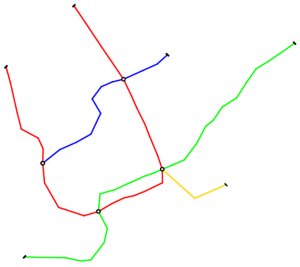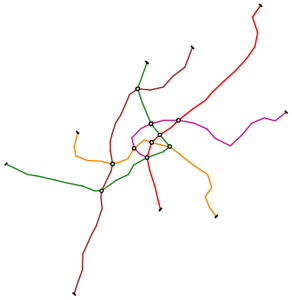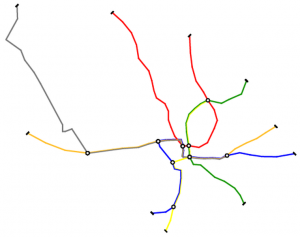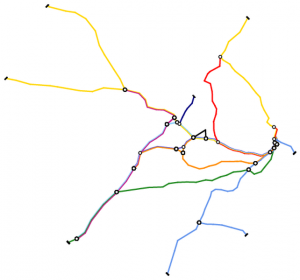# Data-Driven Metro Map Layouts

Generation of schematic maps, e.g. metro maps, can be a time intensive task, when done manually. Automatic generation of such maps enables a designer to explore more versions of the same map, while simultaneously optimizing systematic measures of metro maps, like minimizing the number of bends or the distortion of direction for station connections. Nöllenburg and Wolf presented a Mixed Integer Program for automatic generation of octolinear metro maps, i.e., all edges are horizontal, vertical or parallel to a $$45^\circ$$ diagonal.

We extend this model to incorporate several new features:

• Edges can be parallel to any set of directions. These directions do not need to be axis aligned or evenly spaced.
• The set of directions can be derived based the input map in order to match the available directions as best as possible to the edges in the input.

Some of these features have been presented in an abstract at the informal Schematic Mapping Workshop 2019. This website displays some layouts created by our new framework, using different linearities, orientation systems and objective function weights. Click on the names to see the respective layouts.

 Montreal Vienna Washington Sydney $$65$$ stations $$66$$ edges $$90$$ stations $$96$$ edges $$97$$ stations $$101$$ edges $$173$$ stations $$181$$ edges $$3$$ faces $$4$$ lines $$8$$ faces $$5$$ lines $$6$$ faces $$6$$ lines $$10$$ faces $$10$$ lines## Montreal

Layouts created with objective value weights $$(f_1, f_2, f_3) = (3, 2, 1)$$

Layouts created with objective value weights $$(f_1, f_2, f_3) = (10, 5, 1)$$

Pages: 1 2 3 4 5